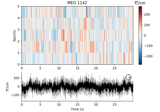# mne.connectivity.envelope_correlation¶

mne.connectivity.envelope_correlation(data, combine='mean', orthogonalize='pairwise', log=False, absolute=True, verbose=None)[source]

Compute the envelope correlation.

Parameters
dataarray_like, shape=(n_epochs, n_signals, n_times) | generator

The data from which to compute connectivity. The array-like object can also be a list/generator of array, each with shape (n_signals, n_times), or a `SourceEstimate` object (and `stc.data` will be used). If it’s float data, the Hilbert transform will be applied; if it’s complex data, it’s assumed the Hilbert has already been applied.

combine‘mean’ | `callable()` | `None`

How to combine correlation estimates across epochs. Default is ‘mean’. Can be None to return without combining. If callable, it must accept one positional input. For example:

```combine = lambda data: np.median(data, axis=0)
```
orthogonalize‘pairwise’ | `False`

Whether to orthogonalize with the pairwise method or not. Defaults to ‘pairwise’. Note that when False, the correlation matrix will not be returned with absolute values.

New in version 0.19.

logbool

If True (default False), square and take the log before orthonalizing envelopes or computing correlations.

New in version 0.22.

absolutebool

If True (default), then take the absolute value of correlation coefficients before making each epoch’s correlation matrix symmetric (and thus before combining matrices across epochs). Only used when `orthogonalize=True`.

New in version 0.22.

verbose

If not None, override default verbose level (see `mne.verbose()` and Logging documentation for more). If used, it should be passed as a keyword-argument only.

Returns
corrndarray, shape ([n_epochs, ]n_nodes, n_nodes)

The pairwise orthogonal envelope correlations. This matrix is symmetric. If combine is None, the array with have three dimensions, the first of which is `n_epochs`.

Notes

This function computes the power envelope correlation between orthogonalized signals 12.

Changed in version 0.22: Computations fixed for `orthogonalize=True` and diagonal entries are set explicitly to zero.

References

1

Joerg F Hipp, David J Hawellek, Maurizio Corbetta, Markus Siegel, and Andreas K Engel. Large-scale cortical correlation structure of spontaneous oscillatory activity. Nature Neuroscience, 15(6):884–890, 2012. doi:10.1038/nn.3101.

2

Sheraz Khan, Javeria A. Hashmi, Fahimeh Mamashli, Konstantinos Michmizos, Manfred G. Kitzbichler, Hari Bharadwaj, Yousra Bekhti, Santosh Ganesan, Keri-Lee A. Garel, Susan Whitfield-Gabrieli, Randy L. Gollub, Jian Kong, Lucia M. Vaina, Kunjan D. Rana, Steven M. Stufflebeam, Matti S. Hämäläinen, and Tal Kenet. Maturation trajectories of cortical resting-state networks depend on the mediating frequency band. NeuroImage, 174:57–68, 2018. doi:10.1016/j.neuroimage.2018.02.018.

## Examples using `mne.connectivity.envelope_correlation`¶